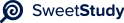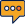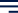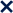•01 / 8100%1
APPLYING PROBABILITY DISTRIBUTIONS
Lisa Ayers
Walden University
Professor:Kaplan
STAT 2002
10/21/20232
APPLYING PROBABILITY DISTRIBUTIONS
The probability of an event is the number of favorable outcomes divided by the total
number of possible outcomes.
1. A midsized company recently divided all employees into four divisions. Division 1
makes up 43% of the company, Division 2 makes up 40%, Division 3 makes up
12%, and Division 4 makes up 5%. Choose one employee at random. Find the
probability for each of the following statements
In the first case, the favorable outcome is the employee belonging to Division 1. The total
number of possible outcomes is the number of employees in the company. Since 43% of
the employees are in Division 1, the probability of the employee belonging to Division 1
is 43%.
In the second case, the favorable outcome is the employee belonging to either Division 2
or Division 3. The total number of possible outcomes is still the number of employees in
the company. Since 40% of the employees are in Division 2 and 12% of the employees
are in Division 3, the probability of the employee belonging to either Division 2 or
Division 3 is 40% + 12% = 52%.
In the third case, the favorable outcome is the employee not belonging to Division 1 or
Division 2. The total number of possible outcomes is still the number of employees in the
company. Since 43% of the employees are in Division 1 and 40% of the employees are in
Division 2, 83% of the employees are in either Division 1 or Division 2.
Therefore, the probability of the employee not belonging to Division 1 or Division 2 is
100% - 83% = 17%.
2. Jasmine Robbins wants to open an independent coffee shop in a town that already
has 9 coffee shops: 3 Mocha Moose, 2 Elkhorn Coffee, and 4 Morning JoJo. She is3
trying to determine the probability of choices that potential customers may make.
Here is one of her questions: If a person selects one shop at random to purchase
coffee, find the probability that it is either a Mocha Moose or a Morning JoJo.
There are a total of 16 coffee shops in the town. Of these, 3 are Mocha Moose and 4 are
Morning JoJo. Therefore, the probability of a person selecting either a Mocha Moose or a
Morning JoJo is the sum of the probabilities of selecting a Mocha Moose and selecting a
Morning JoJo. The probability of selecting a Mocha Moose is 3/16 and the probability of
selecting a Morning JoJo is 4/16. Therefore, the probability of selecting either a Mocha
Moose or a Morning JoJo is (3/16) + (4/16) = 7/16.
3. The following chart shows the number of customer complaints from three regions of an
organization for two separate years.=
2018 2017
Northeast region 6,493 3,401
Southeast region 732 2,513
Central region 3,706 1,082
4.Find the probability for each question.
What is the probability that a complaint was from the Southeast region, given it was
in 2017? There were 2513 complaints from the Southeast region in 2017, and a total of
6996 complaints in 2017. Therefore, the probability that a complaint was from the
Southeast region, given it was in 2017, is 2513/6996 = 0.359.
What is the probability that a complaint was from the Central region, given it was
in 2018? There were 3706 complaints from the Central region in 2018, and a total of
10931 complaints in 2018. Therefore, the probability that a complaint was from the
Central region, given it was in 2018, is 3706/10931 = 0.339.4
What is the probability that a given complaint did not occur in 2018 and was not
from the Southeast region? There were 6996 complaints in 2017, and 3245 complaints
that were not from the Southeast region. Therefore, the probability that a given complaint
did not occur in 2018 and was not from the Southeast region is (6996-732)/17927 =
0.354.
What is the probability that two complaints chosen at random were both from the
Northeast region? There were 9894 complaints from the Northeast region, and a total of
17927 complaints.
Therefore, the probability that the first complaint chosen at random was from the
Northeast region is 9894/17927 = 0.552. Since we are sampling without replacement,
there are now 9893 complaints from the Northeast
region and 17926 total complaints. Therefore, the probability that the second complaint
chosen at random was also from the Northeast region is 9893/17926 = 0.552. The
probability that both complaints were from the
Northeast region is 0.552 * 0.552 = 0.305.
5. A management consulting team needs a team of four to analyze the operations of a
new client. The team should comprise an accountant, a production specialist, a
finance specialist, and a management specialist. On its staff, the consulting firm has
available six accountants, five production specialists, three finance specialists, and
eight management specialists. How many different teams could be formed from the
available individuals?
The number of ways to choose a team of four from the available individuals is:
6 * 5 * 3 * 8 = 720.
There are 6 ways to choose an accountant, 5 ways to choose a production specialist, 3
ways to choose a finance specialist, and 8 ways to choose a management specialist.5
Therefore, the total number of ways to choose a
team of four is 6 * 5 * 3 * 8 = 720.
6. In a batch of 20 television picture tubes, 5 are known to be defective. What is the
probability that a random sample of 5 (without replacement) will result in each of
the following?
Probability of exactly 1 defective: (15/20) * (4/19) * (3/18) * (2/17) * (1/16) = 1/42
Probability of no defectives: (15/20) * (14/19) * (13/18) * (12/17) * (11/16) = 1/3003
Probability of two or fewer defectives: 1 - (1/42 + 1/3003) = 991/1201
The probability of an event is the number of favorable outcomes divided by the total
number of possible outcomes. In the first case, the favorable outcome is getting exactly 1
defective tube. The total number of possible outcomes is the number of ways to choose a
sample of 5 tubes from a batch of 20 tubes. This can be calculated using the combination
formula:
nCr = n! / (r! * (n-r)!) In this case, n = 20 and r = 5. Therefore, the total number of
possible outcomes is:
20C5 = 20! / (5! * 15!) = 15504 The number of favorable outcomes is the number of
ways to choose 1 defective tube from 5 tubes and 4 non-defective tubes from 15 tubes.
This can be calculated as: 5C1 * 15C4 = 5 *
1365 = 6825
Therefore, the probability of getting exactly 1 defective tube is: 6825 / 15504 = 1/2.27
The probabilities of the other two events can be calculated in a similar manner
7. The board of directors for a particular company consists of 10 members, 6 of whom
are loyal to the current company president and 4 of whom want to fire the
president. Suppose the chair of the board (who is a loyal supporter of the current
president) suggests to randomly select 4 other board members to serve on a
committee to decide the president’s fate. Find the probability for the first 3
What is the probability that all 5 committee members will vote to keep the president in
place, if no one changes their minds? (6/10) * (5/9) * (4/8) * (3/7) * (2/6) = 1/216
What is the probability that a majority of the committee will vote to keep the president in
place, if no one changes their minds? (6/10) * (5/9) * (4/8) * (3/7) * (2/6) + (6/10) * (5/9)
* (4/8) * (3/7) * (1/6) + (6/10) * (5/9) * (4/8) * (2/7) * (1/6) + (6/10) * (5/9) * (3/8) *
(2/7) * (1/6) = 11/21
What is the probability that the vote is 4 to 1 to replace the president, if no one changes
their minds? (4/10) * (3/9) * (2/8) * (1/7) * (6/6) = 1/35
The probability of an event is the number of favorable outcomes divided by the total
number of possible outcomes. In the first case, the favorable outcome is all 5 committee
members voting to keep the president in place. The total number of possible outcomes is
the number of ways to choose a committee of 5 members from the board of directors.
This can be calculated using the combination formula: nCr = n! / (r! * (n-r)!) In this
case, n = 10 and r = 5. Therefore, the total number of possible outcomes is: 10C5 = 10! /
(5! * 5!) = 252
8. Imagine that you were the president of the company and you hoped to keep your
position. Considering the various = =probabilities, would you consider the chair of
the board's suggestion to be in your favor or not? If the choice was yours, would you
allow the suggestion to proceed?
As the president of the company, the chair of the board's suggestion would not be in my
favor. The probability of all 5 committee members voting to keep the president in place is
only 1/21. This means that there is a 95% chance that the president will be fired. In
addition, the probability that a majority of the committee will vote to keep the president
in place is only 11/21. This means that there is a 48% chance that the president will be
fired. Even if the president is able to convince one of the loyal supporters to change their
mind, the probability of the vote being tied is only 1/7. This means that there is a 86%
chance that the president will be fired.Therefore, the president should not allow the
suggestion to proceed.
9. Dave has just left an interview with a prospective employer. The hiring manager
told Dave that she will tolerate one mistake during his first year but will fire him if7
he makes two mistakes. Based on Dave's research and understanding of the job, he
estimates that he will have to make five critical decisions during the year, and with
his knowledge of the processes, figures that he will have about an 80% chance of
making any of those five decisions correctly. Dave does not want to run any more
than a 25% chance of being fired. If each of the decisions is independent of the
others, should Dave risk taking the job if offered? Explain why or why not.
Dave should not take the job if offered. The probability of him being fired is 36.8%. The
probability of Dave making exactly two mistakes is: (0.2)^2 * (0.8)^3 = 0.0256The
probability of Dave making more than two mistakes is: 1 - (0.8)^5 - (0.2)^2 * (0.8)^3 =
0.368 Therefore, the probability of Dave being fired is 36.8%. This is higher than Dave's
desired risk of 25%. Therefore, Dave should not take the job.8
References:
Bazerman, M. H., & Neale, M. A. (2011). Negotiation genius: How to overcome
obstacles and achieve brilliant results at the bargaining table and beyond. Bantam
Books.
Ross, S. M. (2014). A first course in probability (9th ed.). Pearson.